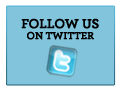Rules of the puzzle: The words are found in all directions - vertically, horizontally, diagonally, forward and backward.
The Solution Phrase: "Follow - Synonyms and Antonyms" (created by the letters that are not used in any word within the puzzle)
To mark a word as found click on the first letter and the last one of the word in the grid below.
 S U C C E E D E C E R P F A S U P E R V E N E S A H C A P R O C E E D I U G O C N P T R A N S I T P I L O T R E T R A V E R S E L M E E C R D I A E L O W W P D D T H A H I G C V S E A A A Y L A M C A S N K R N T T N O U P B R O T R O Y E E A C N S E U A Y U T E R E S U D H E R L E M S R E G E C B S T R O A S A N H S N A D A O R S N T A N D T O E D R N R Y U O E N A V I G A T E D M T S P P

Find these words (For hint click on the word):
 ACCOMPANY, ADHERE, ANTEDATE, ATTEND, CHAPERON, CHASE, COURSE, DISREGARD, GUIDE, IGNORE, NAVIGATE, OBSERVE, PERAMBULATE, PILOT, POSTDATE, PRECEDE, PREDATE, PROCEED, PURSUE, RESULT, SEARCH, SUCCEED, SUPERVENE, TRACE, TRACK, TRANSIT, TRAVERSE, WATCH Next Puzzles
 List of puzzles Who is this painter?(New Word Rebus) Create your own puzzle online Puzzles created by users Puzzle express Contact us